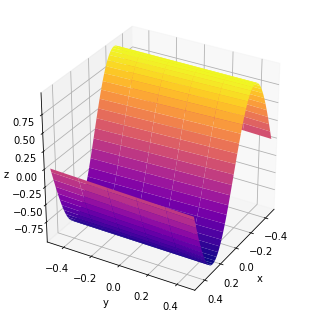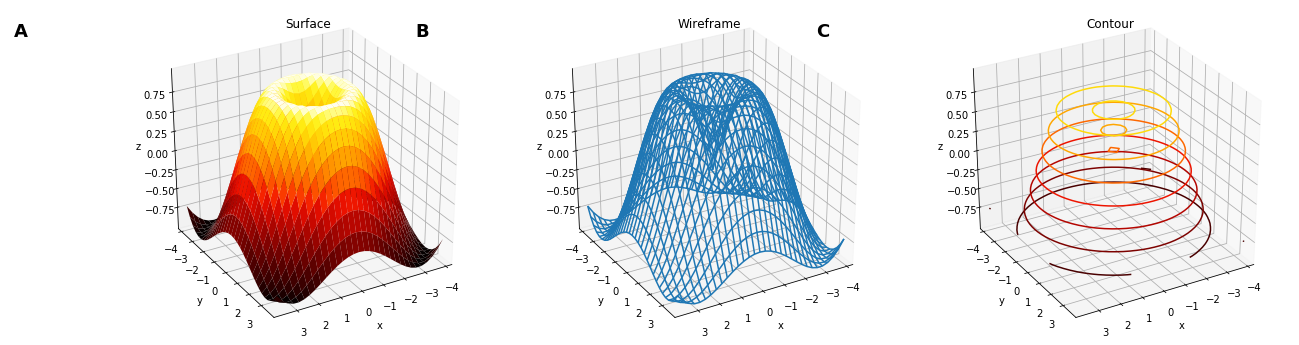Surface¶

Title
Surface Element
Dependencies
Matplotlib
Backends
Matplotlib
In :
import numpy as np
import holoviews as hv
from holoviews import opts

hv.extension('matplotlib')Surface is used for a set of gridded points whose associated value dimension represents samples from a continuous surface. Surface is equivalent to an Image type and supports all the same data formats, including simply NumPy arrays with associated bounds and other gridded data formats such as xarray.

Rendering a large can often be quite expensive, using rstride and cstride we can draw a coarser surface. We can also control the azimuth, elevation and distance as plot options to control the camera angle:

In :
surface = hv.Surface(np.sin(np.linspace(0,100*np.pi*2,10000)).reshape(100,100))

surface.opts(opts.Surface(azimuth=30, elevation=30, rstride=20, cstride=2, cmap='plasma'))
Out:In addition to a simple surface plots, the matplotlib surface plot also supports other related plot_type modes including 'wireframe' and 'contour' plots:

In :
xs = np.arange(-4, 4, 0.25)
ys = np.arange(-4, 4, 0.25)
X, Y = np.meshgrid(xs, ys)
R = np.sqrt(X**2 + Y**2)
Z = np.sin(R)

surface = hv.Surface((xs, ys, Z), label='Surface')
wireframe = surface.relabel('Wireframe').opts(plot_type='wireframe')
contour = surface.relabel('Contour').opts(plot_type='contour')

(surface + wireframe + contour).opts(
opts.Layout(fig_size=150, hspace=0.1),
opts.Surface(azimuth=60, cmap='fire'))
Out:For full documentation and the available style and plot options, use hv.help(hv.Surface).